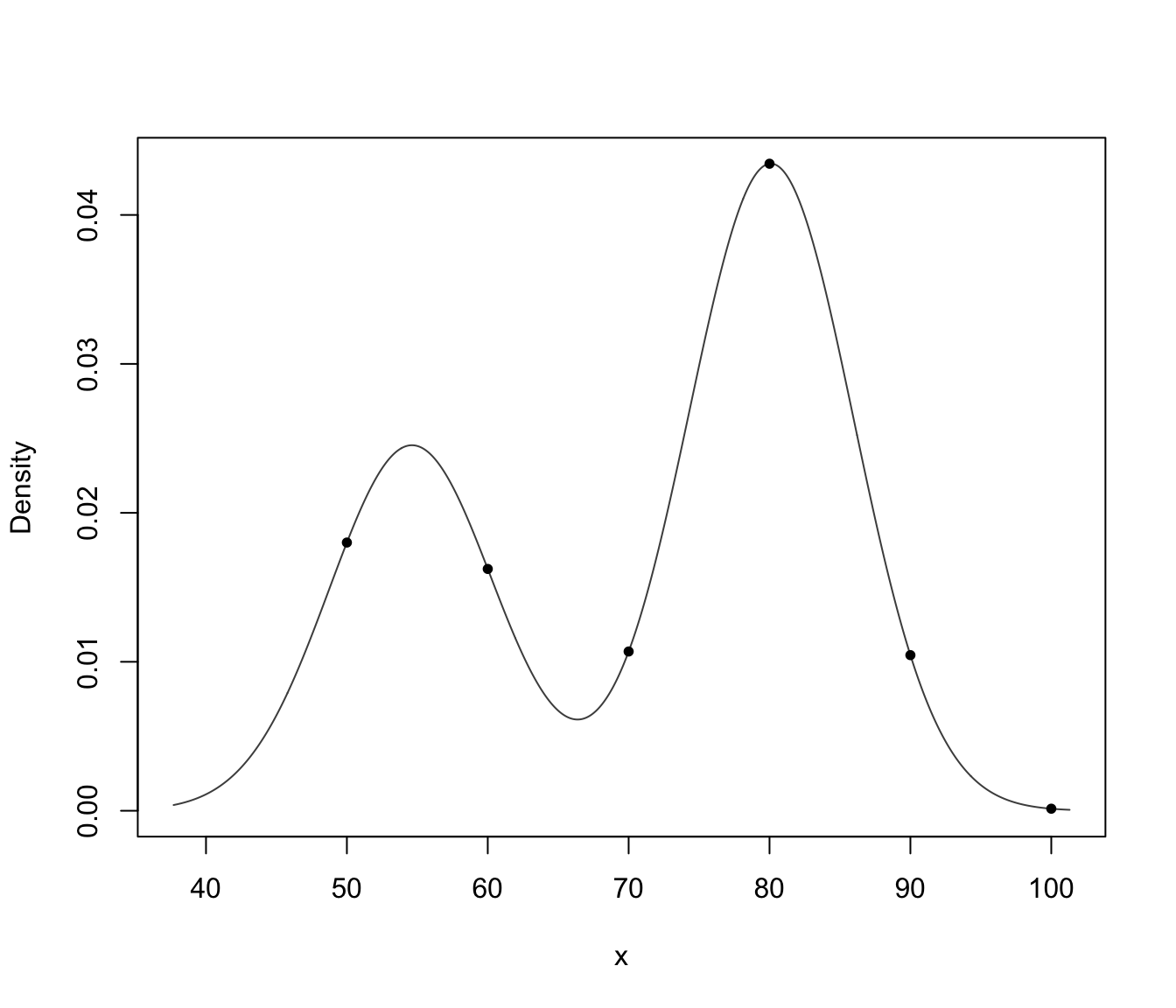Compute density estimation for multivariate observations based on Gaussian finite mixture models estimated by densityMclust.

# S3 method for densityMclust
predict(object, newdata, what = c("dens", "cdens", "z"), logarithm = FALSE, ...)

Arguments

object

an object of class 'densityMclust' resulting from a call to densityMclust.

newdata

a vector, a data frame or matrix giving the data. If missing the density is computed for the input data obtained from the call to densityMclust.

what

a character string specifying what to retrieve: "dens" returns a vector of values for the mixture density; "cdens" returns a matrix of component densities for each mixture component (along the columns); "z" returns a matrix of conditional probabilities of each data point to belong to a mixture component.

logarithm

A logical value indicating whether or not the logarithm of the density or component densities should be returned.

...

further arguments passed to or from other methods.

Value

Returns a vector or a matrix of densities evaluated at newdata depending on the argument what (see above).

Luca Scrucca

Examples

# \donttest{
x <- faithful\$waiting
dens <- densityMclust(x)x0 <- seq(50, 100, by = 10)
d0 <- predict(dens, x0)
plot(dens)points(x0, d0, pch = 20)# }